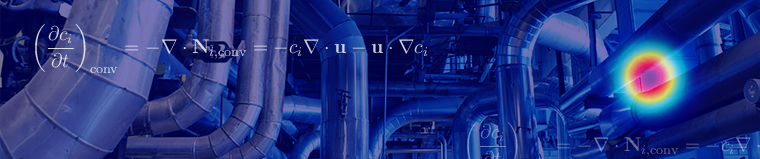## What Is Convection?

Química   O que é Convecção?

### Mass Transfer Due to the Bulk Motion of a Fluid

Convection is mass transfer due to the bulk motion of a fluid. For example, the flow of liquid water transports molecules or ions that are dissolved in the water. Similarly, the flow of air transports molecules present in air, including both concentrated species (e.g., oxygen and nitrogen) and dilute species (e.g., carbon dioxide). Note that the term convection in heat transfer usually refers to the combination of heat transfer by conduction and advection, where advection refers to heat transfer due to bulk fluid motion. In the field of transport phenomena, and also in this text, convection is synonymous with advection.

The animation below illustrates the convection of a region of a fluid, where a certain chemical species is concentrated (indicated by the red color):

Convection of a chemical species (indicated by red coloration) in a flow moving from left to right.

### Describing Convection

The velocity of a molecule undergoing mass transfer incorporates both a convective and diffusive component. We understand convection as mass transport due to the average velocity of all molecules, and diffusion as mass transport due to the instantaneously varying, randomized velocity of individual molecules, compared to the average velocity of the fluid as a whole.

Hence, by a suitable choice of reference velocity, we can identify a diffusive flux and a convective flux. For dilute species transport, where one component, such as a solvent or carrier gas, dominates the momentum of the system, it is normal to take the reference velocity as that of the dominant component.

The motion of a bulk fluid therefore contributes a flux of dilute species, in addition to the flux due to diffusion. This flux can be written as follows:

(1)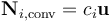where ci is the species concentration (mol m-3) and u is the fluid velocity (m s-1).

The convective flux vector is proportional to the fluid velocity, u, so it acts in the same direction as this velocity. This is because convection is the transport of mass due to bulk fluid motion. For a dilute species, this velocity is the velocity of the solvent or carrier gas. For concentrated species transport, a more careful definition of bulk fluid velocity is required.

The change in concentration at a point, due to convection, follows by substituting the above into the mass continuity equation:

(2)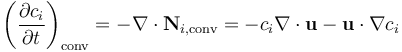The first term (including ∇⋅u) equals zero for an incompressible fluid flow as a result of the conservation of mass of the fluid as a whole. As a consequence, we see that only in the presence of concentration gradients will the concentration at a point be changed by convection:

(3)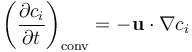This is the convection equation, a time-dependent, first-order partial differential equation.

### Convection as Displacement of a Concentration Profile

A good understanding of the consequences of convection are gained by noting the presence of an exact solution to the convection equation, given a certain initial space-dependent concentration profile, c0(r), and a uniform velocity, u:

(4)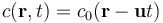Here, we assume that no walls are encountered by the convecting mass. Of course, we do not often encounter exactly uniform flows in reality, but we can often treat flows as locally uniform.

This simple equation illuminates the consequence of convection: The mass of a species, distributed in space according to some concentration profile, is displaced according to the velocity, u. If not, the concentration profile remains unaltered. Thus, in contrast to diffusion, there is no irreversible transport of mass between regions of high and low concentration. In the sense that the flow can be reversed to go in the opposite direction, so can mass transport by convection.

In the images below, we can see convection in action. After 2 seconds of convection, the concentration profile has been displaced by a vector r = u Δt. Since u is 1 m s-1 in the x-direction, this corresponds to a left-to-right displacement of 2 meters.Concentration profile at t = 1 s. Concentration profile at t = 1 s.Concentration profile at t = 3 s. The initial concentration profile is displaced 2 m to the right by convection in the flow. Concentration profile at t = 3 s. The initial concentration profile is displaced 2 m to the right by convection in the flow.

### Convection and Diffusion

Since chemical species have a nonzero diffusivity in reality, it is normal to solve the convection-diffusion equation, where both the diffusive and convective contributions to the mass transport are included:

(5)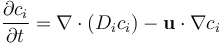A discussion of the combination of the two modes of mass transport, considering the relevant length and time scales, can be found at Convection-Diffusion Equation.

### Natural Convection

Even in the absence of a forced flow, a buoyancy-driven flow may still arise in the presence of temperature gradients because of density variations and the force of gravity. For volumes of fluid larger than a few milliliters under ambient conditions, convection currents normally persist dynamically, without a steady state being attained. This means that convection will, in practice, contribute to mass transport even when no forced convection applies. In the case where density variations are caused by temperature variations, this effect is referred to as natural convection, free convection, or simply buoyant convection.

We encounter this effect when putting sugar into our tea or coffee, where variations in both temperature and composition cause density gradients. Although it is best to induce forced convection by stirring with a spoon, the sugar will still reach a uniform concentration throughout the fluid much more quickly than would be predicted by diffusion theory alone. Another pronounced effect is the convection caused by gradients in salinity in oceans, which is usually referred to as gravitational convection.

### Summary

Convection is mass transport due to bulk fluid motion. Except for microfluidic devices, convection is necessary to achieve effective mass transport because diffusion over human length scales is a very slow process. Most chemical reactors involve some sort of flow and in the case of turbulent flow, mass transport by convection is especially efficient for mixing as well as bulk transport.

While the equations for convection are simple to write down, they cannot be solved by hand, except for the simplest geometries. Numerical modeling is vital to understand the interplay of convection and diffusion in real systems.

Published: January 15, 2015
Last modified: March 21, 2018
Appears In - QUÍMICA